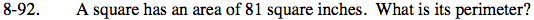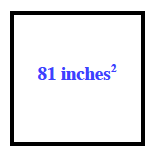### Home > CC1 > Chapter Ch8 > Lesson 8.3.1 > Problem8-92

8-92.

A square has an area of 81 square inches. What is its perimeter? Homework Help ✎Remember that perimeter is the distance around a shape. Also, a square has four sides which are equal in length.

Here is a sketch of a square with an area of 81 square inches. What number multiplied by itself equals 81? That number is the length of one side of the square.